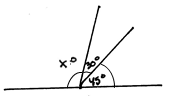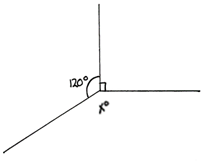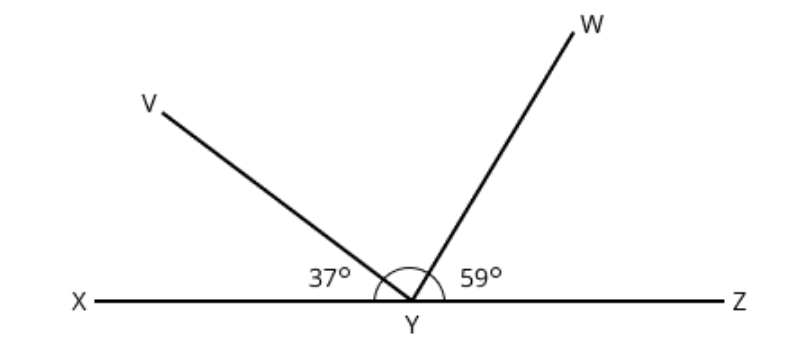# Shapes and Angles

## Objective

Solve problems involving the measure of more than two adjacent angles.

## Common Core Standards

### Core Standards

?

• 4.MD.C.7 — Recognize angle measure as additive. When an angle is decomposed into non-overlapping parts, the angle measure of the whole is the sum of the angle measures of the parts. Solve addition and subtraction problems to find unknown angles on a diagram in real world and mathematical problems, e.g., by using an equation with a symbol for the unknown angle measure.

?

• 1.OA.D.8

## Criteria for Success

?

1. See that angle measure is additive (i.e., when an angle is decomposed into non-overlapping parts, the angle measure of the whole is the sum of the angle measures of the parts).
2. Solve addition and subtraction problems to find unknown angles on a diagram in real-world and mathematical problems that involve more than two adjacent angles, e.g., by using an equation with a symbol for the unknown angle measure (MP.2, MP.4).
3. Write an equation to represent the unknown angle measure in a compound angle (MP.2).
4. Know the angle measure of common angles (a straight angle, a right angle, a full circle) and use that knowledge to solve for missing angle lengths (MP.3).

#### Fishtank Plus

• Problem Set
• Student Handout Editor
• Vocabulary Package

?

### Problem 1

Use patterns blocks of various types to create a design in which you can see a decomposition of 360°.  Which shapes did you use? Write an equation to show how you composed 360°.

#### References

EngageNY Mathematics Grade 4 Mathematics > Module 4 > Topic C > Lesson 11Application Problem

Grade 4 Mathematics > Module 4 > Topic C > Lesson 11 of the New York State Common Core Mathematics Curriculum from EngageNY and Great Minds. © 2015 Great Minds. Licensed by EngageNY of the New York State Education Department under the CC BY-NC-SA 3.0 US license. Accessed Dec. 2, 2016, 5:15 p.m..

Modified by Fishtank Learning, Inc.

### Problem 2

Find the measure of the missing angles in the following figures.

a.b.#### References

EngageNY Mathematics Grade 4 Mathematics > Module 4 > Topic C > Lesson 11Concept Development Problem 1

Grade 4 Mathematics > Module 4 > Topic C > Lesson 11 of the New York State Common Core Mathematics Curriculum from EngageNY and Great Minds. © 2015 Great Minds. Licensed by EngageNY of the New York State Education Department under the CC BY-NC-SA 3.0 US license. Accessed Dec. 2, 2016, 5:15 p.m..

Modified by Fishtank Learning, Inc.
EngageNY Mathematics Grade 4 Mathematics > Module 4 > Topic C > Lesson 10Concept Development Problem 3

Grade 4 Mathematics > Module 4 > Topic C > Lesson 10 of the New York State Common Core Mathematics Curriculum from EngageNY and Great Minds. © 2015 Great Minds. Licensed by EngageNY of the New York State Education Department under the CC BY-NC-SA 3.0 US license. Accessed Dec. 2, 2016, 5:15 p.m..

Modified by Fishtank Learning, Inc.

## Problem Set & Homework

### Discussion of Problem Set

• How does your knowledge of a line assist you in solving #1 and #2?
• How does your knowledge of a circle assist you in solving #3 and #4?
• What was the correct equation in #5 part (a)? How did that help you solve part (b)?
• In #6, what vocabulary term did you need to know to be able to solve?
• What made #7 different from previous problems? How was it similar to and different from some problems in Lesson 12?
• How did #8 differ from the previous problems? How does #8 demonstrate the importance of reading carefully?

?

${\angle XYZ}$  is a straight angle.a.   Write an equation that can be used to solve for the measure of $\angle VYW$.

b.   Find the measure, in degrees, of $\angle VYW$.

#### Mastery Response